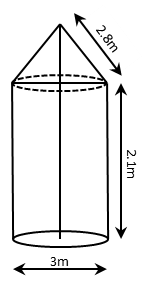# CBSE Class 10 Math Question Paper 2016 | Q12

###### CBSE Maths Board Paper Solution | 3 Mark | Surface Areas of Cones and CylindersClass 10 Maths Questions

The given question is a medium difficulty 3-mark question from the chapter Surface Areas and Volumes. Concept Tested: Computing curved surface areas of a cone and a cylinder. This question appeared in Section C of the 2016 CBSE class 10 board paper.

Question 12: A tent is in the shape of a cylinder surmounted by a conical top of same diameter. If the height and diameter of cylindrical part are 2.1 m and 3 m respectively and the slant height of conical part is 2.8 m, find the cost of canvas needed to make the tent if the canvas is available at the rate of ₹ 500/sq.metre. (Use π = $\frac{22}{7}$.

## NCERT Solution to Class 10 Maths

### Explanatory Answer | CBSE 2016 Maths Paper Q12Cost of Canvas = Area of Canvas × cost per sqm.
The tent is in the shape of a cylinder with a conical top.
Area of Canvas = Curved surface area of cylinder + Curved surface area of cone

##### Step 1: Compute Curved Surface Area of Cylinder

Curved surface area of cylinder = 2 πrh
= 2 × $\frac{22}{7}$ × $\frac{3}{2}$ × 2.1 = $\frac{99}{5}$

##### Step 2: Compute Curved Surface Area of Cone

Curved surface area of cone = πrl
= $\frac{22}{7}$ × $\frac{3}{2}$× 2.8 = $\frac{66}{5}$

Area of Canvas = $\frac{99}{5}$ + $\frac{66}{5}$ = $\frac{165}{5}$ = 33 sqm

##### Step 3: Compute Cost of Canvas

Cost of Canvas = 33 × 500 = ₹ 16,500

###### Try CBSE Online CoachingClass 10 Maths

Register in 2 easy steps and
Start learning in 5 minutes!